Chapter 3 - Coordinate Geometry, Solved Examples, Class 9, Maths

# Chapter 3 - Coordinate Geometry, Solved Examples, Class 9, Maths - Extra Documents & Tests for Class 9

Ex 1. Find the distance between the points P(3, 2) and Q(-4, 5)

Sol. P(3, 2), Q(-4, 5)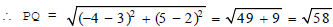Ex 2. Find the point on the x-axis which is equidistance from (2, -5) and (-2, 9).
Sol.
We know that a point on the x-axis is of form (x, 0). Let the point on the x-axis be P(x, 0) and the given points are A(2, -5) and B(-2, 9)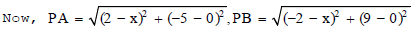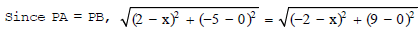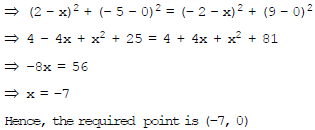Ex 3. In which quadrant do the following points lie ?
(i) (3, 2)

(ii) (-2, 1)

(iii) (-1, -3)

(iv) (5, -1)

Sol. (i) In the point (3, 2) abscissa and ordinate one both positive. So it lies in the 1st quadrant.
(ii) In the point (-2, 1) abscissa is negative and ordinate is positive. So it lies in the second quadrant.
(iii) In the point (-1, -3) abscissa and ordinate are both negative. So it lies in third quadrant.
(iv)In the point (5, -1) abscissa is positive and ordinate is negative. So it ies in the fourth quadrant.

Ex 4. Which of the following points lie on the x-axis.
(i) (1, 1)

(ii) (1, 0)

(iii) (0, 1)

(iv) (0, 0)
(v) (-1, 0)

(vi) (0, -1)

(vii) (4, 0)

(viii) (0, -7)
Sol.
Points of the form (a, 0),i.e. the points in which ordinate is 0, those points lie on the x-axis, and the points in which abscissa is 0, lie on the y-axis.

(ii) (1, 0),

(iv) (0, 0),

(v) (-1, 0),

(vii) (4, 0).

Ex 5. Plot the following points on the graph paper.
(i) A(2, 5)

(ii) B(-5, -7)

(iii) C(3, -2)

(iv) D(0, 5)
(v) E(5, 0)

Sol. Let XOX' and YOY' be the coordinate axes. Then the given points may be plotted as given below :-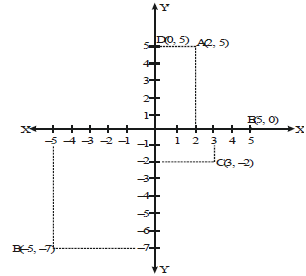Ex 6. Plot the following points on a graph paper.
(i) (3, 4)

(ii) (-2, 3)

(iii) (-5, -2) (iv) (4, -3)
Sol.
Let X'OX and Y'OY be the coordinate axes. The given four points may be potted as given below: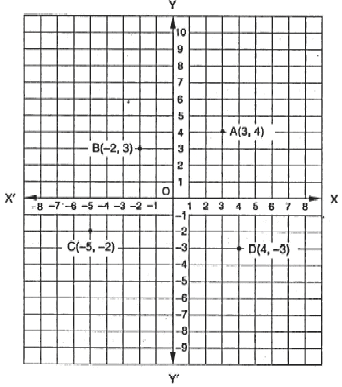Ex 7. From the figure, find :
(i) Absicca
(ii) Ordinate
(iii) Co-ordinate of point P.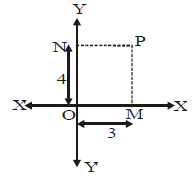Sol. (i) Abscissa = PN = OM = 3 units
(ii) Ordinate = PM =ON = 4 units
(iii) Co-oridinate of the point P = (Abscissa, ordinate) = (3, 4)

Ex 8. Determine y
(i) Abscissa N
(ii) Ordinate
(iii) Co-ordinate of point P given in the following figure.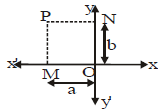Sol. (i) Abscissa of the point P = -NP = -OM = - a
(ii) Ordinates of the point P = MP = ON = b
(iii) Co-ordinates of the point P = (Abscissa, ordinate) = (-a, b)

Ex 9. Write down the
(i) Absicca
(ii) Ordinate
(iii) Co-ordinate of P, Q, R and S as given in figure :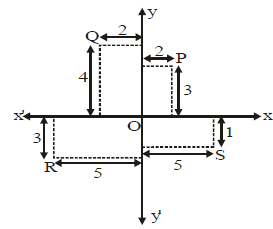Sol. Point P : Abscissa of P = 2 ; Ordinate of P = 3
Co-ordinate of P = (2, 3)
Point Q : Abscissa of Q = -2 ; Ordinate of Q = 4
Co-ordinate of Q = (-2, 4)
Point R : Abscissa of R = -5 ; Ordinate of R = -3
Co-ordinate of R = (-5, -3)
Point S : Abscissa of S = 5 ; Ordinate of S = -1
Co-ordinate of S = (5, -1)

Ex 10. Plot the points A(2, 0), B(2, 2), C(0, 2) and draw the line segment OA, AB, BC and CO. What figure do you obtain ?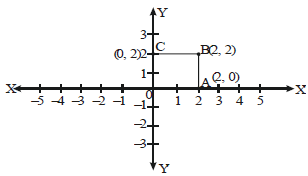Sol. On joining OA, AB, BC and CO, we get a square of each side 2 units.

The document Chapter 3 - Coordinate Geometry, Solved Examples, Class 9, Maths | Extra Documents & Tests for Class 9 is a part of the Class 9 Course Extra Documents & Tests for Class 9.
All you need of Class 9 at this link: Class 9

## Extra Documents & Tests for Class 9

1 videos|228 docs|21 tests

## FAQs on Chapter 3 - Coordinate Geometry, Solved Examples, Class 9, Maths - Extra Documents & Tests for Class 9

 1. What is Coordinate Geometry?Ans. Coordinate Geometry is a branch of mathematics that deals with the study of geometry using the principles of algebra. It involves the study of geometric properties and concepts using coordinate systems, such as points, lines, curves, and shapes.
 2. How is Coordinate Geometry used in real life?Ans. Coordinate Geometry has various real-life applications. It is used in navigation systems to determine the location of a place using latitude and longitude coordinates. It is also used in computer graphics to create and manipulate images, in architecture and engineering to design structures and analyze their dimensions, and in GPS technology to track the movement of vehicles.
 3. What are the different types of coordinates used in Coordinate Geometry?Ans. In Coordinate Geometry, there are mainly two types of coordinates used - Cartesian coordinates and Polar coordinates. Cartesian coordinates use two perpendicular axes, x and y, to represent points in a plane. Polar coordinates, on the other hand, use the distance from the origin and the angle made with a reference line to represent points.
 4. Can you explain the concept of slope in Coordinate Geometry?Ans. In Coordinate Geometry, slope is a measure of the steepness of a line. It is the ratio of the vertical change (change in y-coordinate) to the horizontal change (change in x-coordinate) between two points on the line. The slope of a line can be positive, negative, zero, or undefined, depending on the direction and inclination of the line.
 5. How can we find the distance between two points in Coordinate Geometry?Ans. The distance between two points in Coordinate Geometry can be calculated using the distance formula. The formula is derived from the Pythagorean theorem and is given as: Distance = √((x2 - x1)² + (y2 - y1)²) where (x1, y1) and (x2, y2) are the coordinates of the two points. By substituting the coordinates into the formula, we can find the distance between the points.

## Extra Documents & Tests for Class 9

1 videos|228 docs|21 testsExplore Courses for Class 9 examSignup to see your scores go up within 7 days! Learn & Practice with 1000+ FREE Notes, Videos & Tests.
10M+ students study on EduRev
Track your progress, build streaks, highlight & save important lessons and more!
Related Searches

,

,

,

,

,

,

,

,

,

,

,

,

,

,

,

,

,

,

,

,

,

,

,

,

,

,

,

,

,

,

;